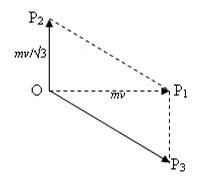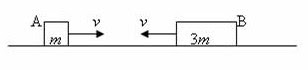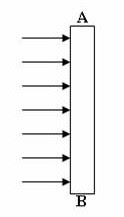## Pages

`“Life is like riding a bicycle.  To keep your balance you must keep moving.”–Albert Einstein`

## Sunday, October 23, 2011

### AP Physics B & C - Multiple Choice Practice Questions on Work, Energy and Power

“The best thinking has been done in solitude. The worst has been done in turmoil.”

– Thomas A. Edison

Today we will discuss a few multiple choice practice questions involving work, energy and power. Questions in this section were discussed earlier on this site. You can access them by clicking on the label ‘work energy power’ or by trying a search for the required word using the search box provided on this page.

(1) A sphere A of mass m moves with speed v and kinetic energy E along the positive x-direction and collides inelastically with another identical sphere B at rest. After the collision sphere A moves with kinetic energy E/3 along the positive y-direction. What is the speed of sphere B after the collision?

(a) v/2

(b) v(√3)/2

(c) v(√5)/2

(d) 2v/√3

(e) 4v/√3

We have E = ½ mv2

Since the kinetic energy is directly proportional to the square of speed, the speed of A after the collision is v/√3.

Momentum is conserved in all collisions (elastic as well as inelastic). The momentum of sphere A before the collision is mv and is directed along the positive x-direction. This is the total momentum of the system since the initial momentum of sphere B is zero.The momentum mv is represented by the vector OP1 in the adjoining figure. The momentum of sphere A after the collision is mv/√3 and is directed along the positive y-direction. This is represented by the vector OP2. The momentum of sphere B after the collision must be given by the vector OP3 since the parallelogram OP2P1P3 is drawn such that its diagonal OP1 represents the final momentum, mv of the system

Evidently OP32 = OP12 + │OP22

Or, OP32 = (mv)2 + (mv/√3)2

But OP3│ = mvf where vf is the speed of sphere B after the collision.

Thus we have

(mvf)2 = (mv)2 + (mv/√3)2

This gives vf = √(v2 + v2/3) = 2v/√3

(2) A particle moves in a plane under the action of a force of constant magnitude. If the direction of the force is always at right angles to the direction of motion of the particle, it follows that

(a) the momentum of the particle is constant

(b) the velocity of the particle is constant

(c) the kinetic energy of the particle is constant

(d) the acceleration of the particle is constant

(e) the particle moves along a straight line with increasing speed

A force of constant magnitude acting always at right angles to to the direction of motion of the particle will supply centripetal force required for uniform circular motion. In uniform circular motion the speed of the particle is constant.

[The velocity is not constant since the direction changes continuously. The momentum and the acceleration also are not constant for the same reason].

Since the speed is constant, the kinetic energy of the particle is constant [Option (c)].

(3) A ball of mass 0.5 kg is thrown vertically downwards from the edge of a building of height 65 m. The initial speed of the ball is 20 ms–1. If the ball strikes the ground with a speed of 40 m/s, the energy lost by the ball because of air resistance is (gravitational acceleration, g = 10 ms–2)

(a) 10 J

(b) 25 J

(c) 30 J

(d) 40 J

(e) 45 J

The initial energy of the ball is Ei = mgH + ½ mvi 2

The first term is the gravitational potential energy of the ball of mass m at height H and the second term is the initial kinetic energy of the ball having initial speed vi. Substituting proper values, we have,

Ei = 0.5×10×65 + ½ ×0.5×202 = 325 + 100 = 425 J.

The final energy of the ball (at the ground level) having velocity vf is Ef = ½ mvf2

Substituting proper values, we have,

Ef = ½×0.5×402 = 400 J.

Therefore, the energy lost by the ball because of air resistance is Ei Ef = 425 J 400 J = 25 J.(4) Two blocks A and B of masses m and 3m respectively (Fig.) travel in opposite directions with the same speed v along a smooth surface. They collide head-on and stick together. Mechanical energy lost during the collision is

(a) ½ mv2

(b) mv2

(c) (3/2) mv2

(d) 2 mv2

(e) 3 mv2

Total momentum of the system before collision is 3mvmv = 2 mv

[We have taken the leftward momentum as positive and that’s why the total momentum is positive. If you take the leftward momentum as negative, the total momentum will be negative. But this won’t affect your final answer and the energy lost during the collision will be positive].

Since the momentum of the system is conserved, the total momentum after the collision will be 2 mv itself. If the common velocity of A and B (which stick together) after collision is vf, we have,

(m + 3m)vf = 2 mv

Therefore vf = v/2

The initial kinetic energy of the system is ½ mv2 + ½ (3m)v2 = 2 mv2

Final kinetic energy of the system is ½ (4m)vf2 = ½ (4m)(v/2)2 = ½ mv2

Therefore, the loss of kinetic energy during the collision = 2 mv2 ½ mv2 = (3/2) mv2

(5) An electric motor creates a tension of 1000 N in a hoist cable and reels it at the rate of 2.5 ms–1. The power output of the motor is

(a) 250W

(b) 400 W

(c) 2.5 kW

(d) 2500 kW

(e) 400 kW

Since the point of application of the force of 1000 N moves through 2.5 m per second, the work done per second (which is the power output) is 1000×2.5 watt = 2500 watt = 2.5 kW

## Sunday, October 9, 2011

### AP Physics B - Multiple Choice Practice Questions on Geometric Optics

"I know not with what weapons World War III will be fought, but World War IV will be fought with sticks and stones."

–Albert Einstein

Questions on optics were discussed on earlier occasions on this site. You can access those questions by clicking on the label ‘optics’ below this post. Generally simple questions will be asked from this section. But to answer them correctly you need to have a thorough knowledge of basic things in this section.

Today we will discuss a few more multiple choice practice questions on geometric optics (geometrical optics):

(1) A thin converging lens produces an inverted real image of an object on a screen. The size of the image is the same as that of the object. When the upper half of the lens is covered by an opaque sheet of paper,

(a) the image disappears

(b) the lower half of the image disappears

(c) the upper half of the image disappears

(d) full image with reduced intensity is obtained

(e) full image having half the size of the object is obtained

Even a small portion of the lens can produce the full image of the object. But the brightness (intensity) of the image will be reduced since half the aperture of the lens admits light. The correct option is (d).

(2) A lens behaves as a converging lens in air and in a liquid of refractive index n1. But it behaves as a diverging lens in liquids of refractive indices n2 and n3. The refractive index of the material of the lens is

(a) greater than both n2 and n3

(b) less than n1 but greater than n2

(c) less than n3 but greater than n2

(d) greater than n1 but less than both n2 and n3

(e) less than n1 but greater than both n2 and n3

The refractive index of the material of the lens must be less than both n2 and n3 for the convergent lens to become divergent. Since the lens continues to be convergent in the medium of refractive index n1, the refractive index of the material of the lens must be.greater than n1. This follows from lens maker’s equation,

1/f = [(n’/n) – 1] (1/R1 – 1/R2) where ‘f’ is the focal length of the lens, n’ is the refractive index of the material of the lens, n is the refractive index of the medium in which the lens is placed and R1 and R2 are the radii of curvature of its surfaces. The quantity (1/R1 – 1/R2) is positive in the case of a lens that is converging in air. The ratio n’/n has to be greater than 1 for the focal length to be positive (and hence the lens to be converging) when the lens is placed in any other medium.

[A simple ray diagram will lead to the answer to the above question. The adjoining figure shows the manner in which a lens which is converging in air becomes diverging in a medium of refractive index greater than that of the lens. At the first surface of the lens, the ray is refracted away from the normal where as at the second surface the ray is refracted towards the normal. The net effect is to bend the ray further away from the principal axis of the lens].

(3) A thin monochromatic beam of light proceeding through glass is incident at an angle i at glass-air interface. The beam is partially reflected and partially refracted at the interface such that the angle between the reflected and refracted beams is 90º, as shown in the figure. If the angles of reflection and refraction are r and r respectively, what is the critical angle for the glass-air interface?.

(a) sin–1 (r/r’)

(b) sin–1(r–r’)

(c) sin–1(tan r)

(d) sin–1(cos r)

(e) sin–1(tan r’)

The critical angle C and refractive index n of glass with respect to air is given by

n = 1/sin C ………….(i)

here n = sin r/sin i = sin r/sin r since i = r

Since it is given that the angle between the reflected and refracted rays is 90º, we have (with reference to the figure) r = (90º – r)

Therefore, n = sin r/sin r = sin(90º – r)/sin r =cos r/sin r = 1/tan r

Substituting in Eq (i), we have

1/tan r = 1/sinC

Or, sin C = tan r so that C = sin–1(tan r)(4) A transparent slab with parallel faces has its refractive index increasing linearly with distance from end A to end B (Fig.). Parallel rays of light are incident normally on this slab as shown. What will happen to these rays? (Ignore reflection at the face of the slab).

(a) They will become convergent after refraction

(b) They will become divergent after refraction

(c) They will bend towards A consequent on refraction

(d) They will bend towards B consequent on refraction

(e) They will proceed undeviated.

Since the angle of incidence is zero (normal incidence), the angle of refraction also is zero irrespective of the refractive index. Therefore the rays will proceed undeviated.(5) The figure shows five lenses a, b, c, d, and e made of glass. When placed in air which lens/lenses will act as converging lens/lenses?

(a) None

(b) a, c and d

(c) d only

(d) a and d

(e) c and d

Lens (d) is a planoconvex lens and is converging. Lens (c) has one surface convex and the other concave. But the convex surface has smaller radius of curvature. Therefore, the divergence produced because of the concave surface is more than compensated by the convergence produced by the convex surface. It can therefore function as a converging lens.

Lens (a) also has convex and concave faces. But the radius of curvature of the concave face is smaller so that the convergence produced by the convex face is more than compensated by the divergence produced by the concave face. It will act as a diverging lens.

Lenses (b) and (e) are evidently diverging lenses.

So (c) and (d) are the converging lenses [Option (e)].

## Monday, October 3, 2011

### AP Physics B – Answer to Free Response Practice Question on Fluid Mechanics

He who joyfully marches in rank and file has already earned my contempt. He has been given a large brain by mistake, since for him the spinal cord would suffice.
Albert Einstein
In the post dated 30th September 2011 a free response practice question on fluid mechanics was given to you. As promised, I give below a model answer along with the question:The adjoining figure shows an empty thin walled cubical vessel of side 0.4 m and mass 6.4 kg floating on kerosene contained in a large tank (not shown). Density of kerosene is 800 kgm–3 where as the density of water is 1000 kgm–3. You may take the acceleration due to gravity as 10 ms–2. Now, answer the following questions:
(a) Calculate the magnitudes of the force of buoyancy and the force of gravity acting on the empty vessel and state their directions.
(b) Calculate the height x of the portion of the empty vessel that is submerged in kerosene.
(c) Water is slowly poured into the vessel so that an additional 0.2 m of the height of the vessel is submerged in kerosene. Calculate the volume of water added to obtain this condition.
(a) The force of buoyancy acts vertically upwards while the force of gravity (which is the weight of the vessel) acts vertically downwards. They have the same magnitude since the vessel is in equilibriumn. Since the mass (m) of the empty vessel is 6.4 kg, its weight (mg) is 6.4×10 = 64 newton.
Therefore the force of buoyancy and the force of gravitaty have the same magnitude of 64 N.
(b) The weight of a floating body is equal to the weight of the displaced liquid (in accordance with the law of floatation). Therefore we have
Axρg = mg where A is the area of the base of the vessel, x is the height of the portion of the empty vessel that is submerged in kerosene, ρ is the density of kerosene and g is the gravitational acceleration.
This gives x = m/Aρ = 6.4/(0.42×800) = 0.05 m
(c) When an additional 0.2 m of the vessel is submerged in kerosene, the additional volume of kerosene displaced by the vessel is 0.2 A and the weight of this volume of kerosene is 0.2 Aρg. This must be equal to the weight of the water added so that we have
0.2 Aρg = Vdg where V is the volume of water added.and d is the density of water.
This gives V = 0.2 Aρ/d = (0.2×0.42×800)/1000 = 0.0256 m3
[Suppose you were asked to determine the pressure exerted by water at the bottom of the vessel when the condition mentioned in part (c) in the above question is attained. You will then divide the weight of the water by the area of the bottom of the vessel, remembering that the thrust at the bottom is produced by the weight of the water column in the vessel and pressure is thrust per unit area.
Thus presuure at the bottom = 0.2 Aρg/A =0.2 ρg = 0.2×800×10 = 1600 pascal
(This is the same as Vdg/A)].

Now, go over here to find some more refreshing questions in this section.